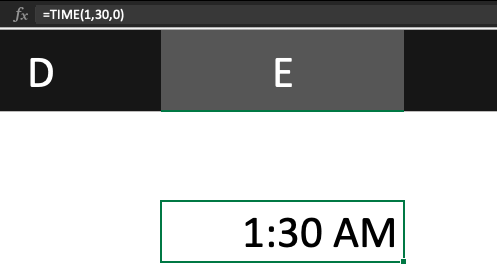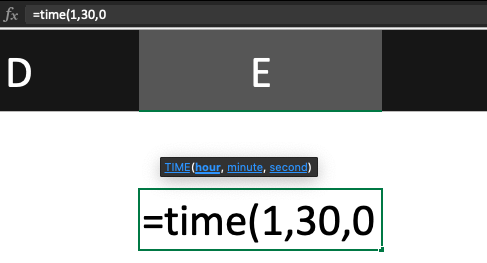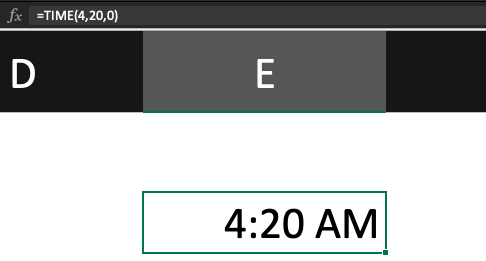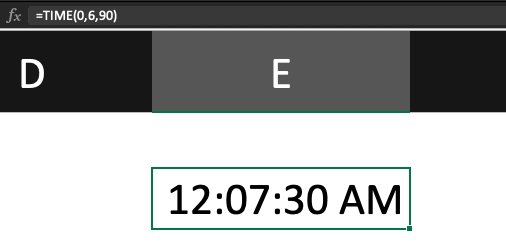# TIME Function

A function in excel that returns the time of day given hour, minute, and second values.Reviewed by

Christy Grimste

Expertise: Real Estate | Investment Property Sales

Updated:

November 25, 2023

## What is the TIME Function?

Excel's TIME function returns the time of day given hour, minute, and second values. The return time is formatted as a time value. The TIME function is for ensuring that time values are entered correctly.

It takes three arguments and returns a time value in the format HH: MM: SS. For example, if the current time is 12:00:00, the function will return to 12:00:00.

Where,

• HH = Hours
• MM = Minutes
• SS = Seconds

To use the time function, enter = time() into a cell. This will insert the current system time into the cell. You can then format the cell as a time value to display the time in the desired format.

The time function is an incredibly useful tool. Depending on the type of data you’re working with, this function can act as the keystone to your worksheets. Therefore, understanding how it works and when to use it will be an important asset in your excel tool portfolio.

## How to use the TIME Function in Excel?

In Excel it is a built-in function that allows you to work with time values. TIME is useful when you need to combine values from different cells or when you need to convert a time value into a different format.

The function can be used to track the amount of time that passes between two events. Often used to track the duration of events, such as how long a person takes to complete a task.

To use the time function, you will need to enter two-time values into two separate cells. The function will then calculate the amount of time between the two events.

A time function is a valuable tool for businesses that need to track employee productivity or project timelines. By tracking the amount of time between two events, businesses can identify areas where they can improve efficiency.

It is important because it can help avoid errors when working with time values. For example, if you try to enter a time value into a formatted cell as a date, you will get an error.

However, it converts the time value into a date format; the cell will accept the value without any errors.

Overall, it is a valuable tool that can help you work with time values in Excel. Whether converting time values into different formats or combining values from different cells, TIME can help you get the job done.

## TIME Function Formula

The time function in Excel syntax is as follows:

=time(hours, minutes, seconds)

This function will take the given hours, minutes, and seconds and convert them into a time value. For example, if you enter the following into a cell:= time(1,30,0)
The cell will display the time 1:30:00.Here you can see =time(1,30,0) is converted to 1:30 am.For the hour section, anything greater than 23 will be divided by 24, and the remainder will be interpreted as the hour value, which you can see in the last example. Similarly, anything greater than 59 will be divided by 60 and be interpreted as an hour value.

Numbers greater than 59 for the second's section will be divided by 60 and converted to a minute value.

And that’s it. The time function is a supremely useful and simple function that can help you keep track of times accurately within the context of your worksheet.

## TIME Function Examples

Below are some pertinent examples of how the time function might look like in one of your cells. It’s relatively straightforward, but hopefully, the illustrations can help you implement this function quicker and more effectively.

As we discussed, the syntax is =time(hours, minutes, seconds). As such, you’ll enter the desired hour, minute, and second values to deliver the time you want in the cell.

For example:

TIME(4,20,00) = 04:20:00 AMTIME(0,06,90) = 12:07:30 AMThe time function is important in excel because it helps you track changes over time. This is especially useful when you are working with constantly changing data, such as stock prices.

Using this function, you can track how your data changes over time and make better decisions about when to buy or sell.

There is also a myriad of other uses for this function that you can employ. Excel’s ability to store and manipulate both dates and times is one of its most powerful features. By understanding the time function, you unlock a world of possibilities in how you structure your workflow.### Everything You Need To Build Your Accounting Skills

To Help You Thrive in the Most Flexible Job in the World.

Researched and authored by Jake Heimowitz | LinkedIn